# GMAT Quant Questions - Problem Solving & Data Sufficiency

GMAT Q-51 Practice / All questions

Must solve 100 GMAT practice questions. The categorization of the type of question and the concept tested are listed in the right column for each question. Detailed explanatory answers, solution videos and slide decks are also provided.

• 1
• If x is a positive integer such that (x-1)(x-3)(x-5)....(x-93) < 0, how many values can x take? A. 47
B. 23
C. 46
D. 21
E. 22
• Problem Solving
• Positive & Negative numbers
• Odd & Even numbers
x can take 23 integer values.

Solution

• 2
• Is x3 > x2 Statement 1: x > 0
Statement 2: x < 1
• Data Sufficiency
• Exponents
Statement (2) alone is sufficient while statement (1) is not.

Solution

• 3
• If m and n are non-zero integers, is mn > nn? Statement 1: |m| = n
Statement 2: m < n
• Data Sufficiency
• Exponents
• Absolute Value
Statement(1) alone is sufficient; statement (2) is not.

Solution

• 4
• If two distinct integers a and b are picked from {1, 2, 3, 4, .... 100} and multiplied, what is the probability that the resulting number has EXACTLY 3 factors? A.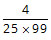B.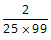C.D.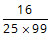E.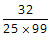Solution

• 5
• What is the remainder when the positive integer x is divided by 6? Statement 1: When x is divided by 7, the remainder is 5.
Statement 2: When x is divided by 9, the remainder is 3.
• Data Sufficiency
• Number Properties
Statements (1) and (2) TOGETHER are NOT sufficient to answer the question asked, and additional data specific to the problem are needed.

Solution

• 6
• Working alone, A can complete a task in ‘a’ days and B in ‘b’ days. They take turns in doing the task with each working 2 days at a time. If A starts they finish the task in exactly 10 days. If B starts, they take half a day more. How long does it take to complete the task if they both work together? A.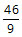B.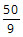C.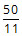D.E.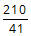• Algebra
• Problem Solving
• Work Time

Working together they will takedays.

Solution

• 7
• A candy manufacturer decided to decrease the weight of each candy bar, while retaining the price. By how many cents did the per kilogram cost of candy change after the reduction in weight? Statement 1: The weight of each piece of candy bar reduced by 9 grams.
Statement 2: The weight of each piece of candy bar reduced by 9%

Solution

• 8
• In the figure given below, ABC and CDE are two identical semi-circles of radius 2 units. B and D are the mid points of the arc ABC and CDE respectively. What is the area of the shaded region?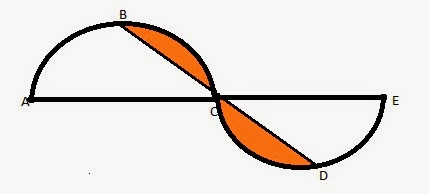A. 4π - 1
B. 3π - 1
C. 2π - 4
D. ½(3π - 1)
E. 2π - 2
• Problem Solving
• Geometry
• Semicircles
• Isosceles Triangles
The area of the shaded region is
(2π - 4) sq units.

Solution

• 9
• a, b, and c are sides of a right triangle. What is the area of the triangle? Statement 1: a = 4.
Statement 2: a + b + c = 4.
• Geometry
• Data Sufficiency
• Right Triangles
Statements (1) and (2) TOGETHER are sufficient to answer the question asked.

Solution

• 10
• If a, b, and c are not equal to zero, what is the difference between the maximum and minimum value of S? $$S = 1+\frac { \left| a \right| }{ a } +\frac {2 \left| b \right| }{ b } +\frac {3 \left| ab \right| }{ ab } -\frac {4 \left| c \right| }{ c } \\$ A. 12 B. 14 C. 22 D. 20 E. 18 • Problem Solving • Algebra • Absolute Value • Correct AnswerChoice$E).
The difference between the maximum and minimum value is 18.

Solution

• 11
• Consider a set S = {2, 4, 6, 8, x, y} with distinct elements. If x and y are both prime numbers and 0 < x < 40 and 0 < y < 40, which of the following MUST be true? I. The maximum possible range of the set is greater than 33.
II. The median can never be an even number.
III. If y = 37, the average of the set will be greater than the median.

A. I only
B. I and II only
C. I and III only
D. III only
E. I, II, and III

Solution

• 12
• If x and y are integers and |x - y| = 12, what is the minimum possible value of xy?
A. -12
B. -18
C. -24
D. -36
E. -48
• Problem Solving
• Algebra
• Absolute Value
The minimum possible value for xy is -36.